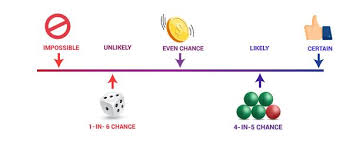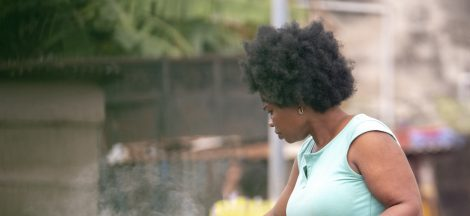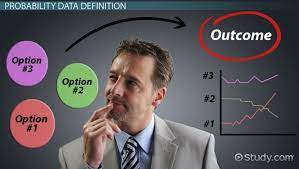We live in a world of uncertainty. No one knows for sure if flipping a coin will first result in a head or a tail. Neither are we certain who will win or lose an election or whether it will or will not rain next week. The chance of either option is at best 50-50. But probability measures the likelihood of each outcome to draw us close to a degree of certainty. Thus, it is the study of chance.

The objective of every business is to maximise profitability. Informed decision making is critical for successful business outcomes. Probability is the mathematical concept that allows predictions to be made in the face of uncertainty. Information from probability is then used by businesses to plan and predict future occurrences.

Here are some basic rules and methods of probability:

Basic Probability Rules

First, the probability of all possible outcomes of an event or trial is 1 or 100%. Thus, the probability of an event happening lies somewhere between 0 and 1, where 0 is unlikely and 1 is likely to happen. Therefore, for any given event A, the range of possible probabilities lies between 0 and 1, mathematically represented as 0 ≤ P(A) ≤ 1.

Simply put, a probability close to 1 means the event is almost certain to happen and close to 0, it is unlikely to happen. When the probability is 0.5, it implies that it is neither likely nor unlikely to happen, it is a 50% of happening or not.

Assigning Probabilities

Second, when thinking of probability, there are three ways in which it is assigned to an outcome. They include:

1.The Classical method: refers to assigning equal probabilities to each likely outcome i.e.,1 /n (where n is the total possible outcomes). A good example is rolling a dice where possible outcomes are six (1,2,3,4,5,6). Here, the probability of each sample point is 1/6. Adding all the probabilities together will result in 1.

2. Relative frequency method: based on experimental and historical data. Relative frequency is the ratio of the occurrence of one event and the total number of possible outcomes. This is calculated by dividing the individual frequencies of an item by the total number of repetitions that has occurred.

3. The Subjective method: this method assigns probability based on sound judgement and experience. It relies on the degree of belief a statistician has in the occurrence of an event. It is used by experienced professionals.

Learning experience

The introduction to probability taken by Dr. Bongo Adi brought to light the fact that we apply probability in everyday life. For every time we consider the possibility of the outcome of an event, we are unknowingly applying the concept of probability. Practical exercises done in the classroom enabled a deeper understanding of probability and its application in the corporate world. I recently applied this knowledge in solving the ACE Automobile case study in the analysis of business problems module.

Conclusion

Finally, the level of uncertainty in the world is enormous. Probability is that mathematical concept that attempts to quantity it. In doing so, it provides useful information on the degree of certainty of possible outcomes of an event. This critical information can form the basis for making informed business decisions and future predictions.

#EMBA27 #MBA Chronicles## Next Generation Fixed Wireless Access (NGFWA) for Service Providers: A Viable Alternative to Traditional Fiber-Optic Networks

in## Transforming Nigeria’s Local Government System for Rural Economic Growth and Increased GDP

in